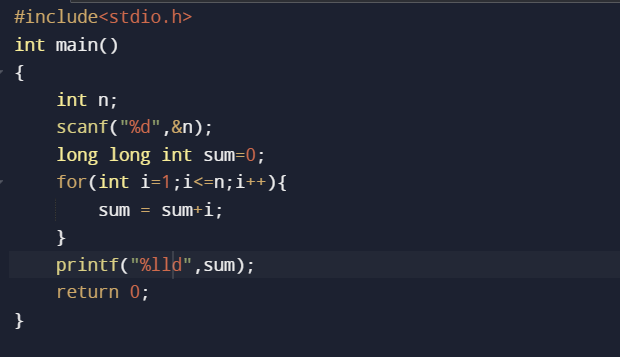# Check code

Hello there,
I’m trying to solve a easy question but I can’t solve it , I can’t figure it out.

code :

``````#include <stdio.h>

int main () {
int n;
scanf("%d",&n);
long long int sum = 0;
for (int i=1; i<=n;i++) {
sum = sum+i;
}
printf("%d",sum);
return 0;
}

``````

As you use %d as a format specifier for printing the sum of all natural numbers. this will be execute very well but there is a limit when the sum goes up from 65535 so the output will come in negative values
so by changing the format specifier to %lld from %d so it will give you the correct answer

in simple words, you use the wrong format specifier so use %lld you will get the output
as you can see the correct code in this picture